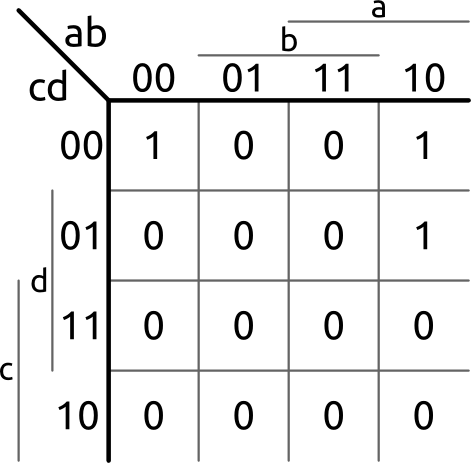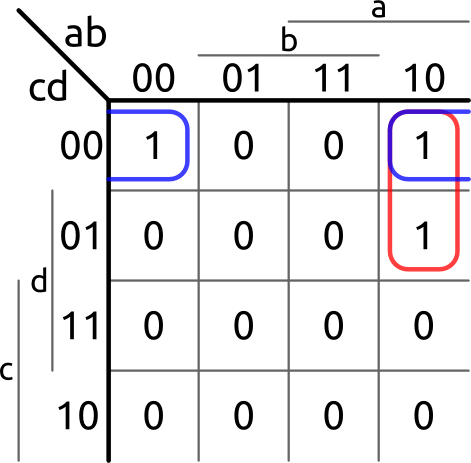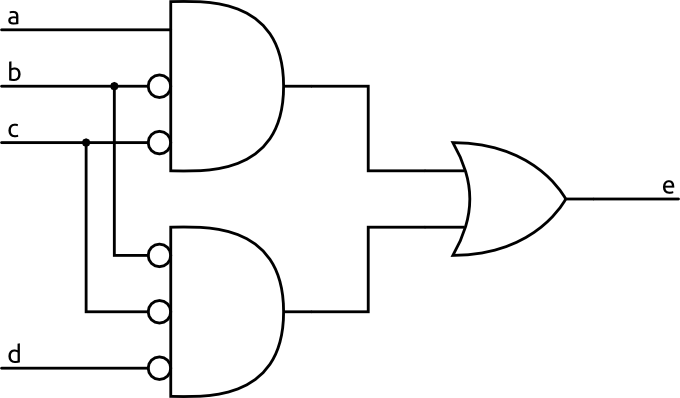# Combinational Logic

There are two main types of digital circuits. The first, and the one we are covering here, is called combinational logic. For a circuit to be considered combinational, it's output must be the same for a given input no matter when that input is applied. An example of this is an addition circuit. No matter when you apply the inputs 2 and 4 the output will always be 6. Because of this requirement, combinational circuits almost never have feedback.

Here is an example.#### Truth Tables

To be able to tell what a circuit does, it is often very helpful to write out a truth table. What is a truth table? A truth table is simply a table that tells you the output of a circuit for every given input.

Here is this circuit's truth table.

Input a Input b Input c Input d Output e
0 0 0 0 1
0 0 0 1 0
0 0 1 0 0
0 0 1 1 0
0 1 0 0 0
0 1 0 1 0
0 1 1 0 0
0 1 1 1 0
1 0 0 0 1
1 0 0 1 1
1 0 1 0 0
1 0 1 1 0
1 1 0 0 0
1 1 0 1 0
1 1 1 0 0
1 1 1 1 0

It is easy to see now that there are only three cases when the circuit outputs a 1.

To create a table like this for basic circuits, you can just apply the inputs in your head or write down the intermediate values as well. Another approach that I like to use is to figure out what inputs cause a 1 and then you know everything else has to be a 0. For example, in this circuit I know that b and c must be 0 for the output to be 1. In the few cases where b and c are 0, a has to be 1 or d has to be 0. After marking those three cases with a 1, you can mark everything else with a 0 to complete the table.

Truth tables are often useful, not just for analyzing circuits, but actually creating them. If you know what function you would like to implement you can write it out in a truth table which can be used to create the circuit.

#### Karnaugh Maps

You may be thinking, there seem to be many ways to implement a truth table, how do I know which is best? Don't worry! There's a tool created specifically to simplify circuits. This tool is the Karnaugh map, also known as K-map.

A Karnaugh map is basically just a reorganization of the truth table.The important thing to notice here is how the table is setup. It is crucial that when moving to any adjacent box only one input can change. For example, if you look at the box where ab = 01 and cd = 01, you can see that moving up changes d, moving down changes c, moving left changes b, and moving right changes a.

The lines on the sides are there just to help make it more clear. The boxes where the lines are are where the input is 1. For example, in the bottom right four boxes, where the c and a sections overlap, c and a are both 1.

To simplify the circuit you just have to group the 1's together into pairs of 2.You can see that you are allowed to wrap around the map to the other sides to group the 1s as well. If there are multiple groups of 1s together then you can combine them into groups of 4, 8, or 16. You can't group them in any groups that are not multiples of 2. The larger the groups you are able to make the simpler the circuit.

In this case we have two groups. Those groups are called implicants. In this example there are no redundant implicants so these two are also the prime implicants. It is possible to have a circuit where you can have unnecessary implants. All that is important is that you cover each 1 with at-least one implicant. If you can remove an implicant while still covering all the 1s then it is redundant.

The implicants for this circuit are ab'c' and b'c'd' where the ' means not. For the first implicant you can see that we were able to drop d out of it because the output is one regardless of what d is when a, b, and c match the implicant. The same goes for a in the second implicant. The larger the implicant the more terms you can drop.

To create a circuit from the implicants you simply or them all together.This circuit performs the same function as the original. You can verify this by writing out a truth table for it.

A circuit of this form is known as a sum-of-products circuit. That's because when bits are anded that is sometimes shown as multiplication and when they are ored it is shown as addition.

It is arguable that this circuit is no simpler than the one we started with and it is true that with only a few minor changes you actually end up with the same circuit. However, the techniques still hold and work well for more complex circuits, or for just creating them.

Karnaugh maps, while useful for basic circuits, get really messy for circuits with more than 4 inputs. You have to start moving into more than 2 dimensions. Luckily there are tools that will simplify circuits for you, so you only need to specify the circuits behavior.